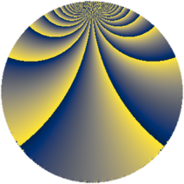# Properties

 Label 162.3.hLevel $162$ Weight $3$ Character orbit 162.h Rep. character $\chi_{162}(5,\cdot)$ Character field $\Q(\zeta_{54})$ Dimension $324$ Newform subspaces $1$ Sturm bound $81$ Trace bound $0$

# Related objects

## Defining parameters

 Level: $$N$$ $$=$$ $$162 = 2 \cdot 3^{4}$$ Weight: $$k$$ $$=$$ $$3$$ Character orbit: $$[\chi]$$ $$=$$ 162.h (of order $$54$$ and degree $$18$$) Character conductor: $$\operatorname{cond}(\chi)$$ $$=$$ $$81$$ Character field: $$\Q(\zeta_{54})$$ Newform subspaces: $$1$$ Sturm bound: $$81$$ Trace bound: $$0$$

## Dimensions

The following table gives the dimensions of various subspaces of $$M_{3}(162, [\chi])$$.

Total New Old
Modular forms 1008 324 684
Cusp forms 936 324 612
Eisenstein series 72 0 72

## Trace form

 $$324 q + O(q^{10})$$ $$324 q + 72 q^{18} + 108 q^{20} + 270 q^{21} + 162 q^{23} - 54 q^{27} - 162 q^{29} - 216 q^{30} - 378 q^{33} - 486 q^{35} - 180 q^{36} - 108 q^{38} + 216 q^{41} + 432 q^{45} + 324 q^{47} + 126 q^{51} - 216 q^{57} - 378 q^{59} - 540 q^{63} - 1728 q^{65} - 1008 q^{66} + 702 q^{67} - 216 q^{68} - 1872 q^{69} + 540 q^{70} - 1296 q^{71} - 576 q^{72} - 864 q^{74} - 900 q^{75} + 108 q^{76} - 864 q^{77} - 288 q^{78} + 108 q^{79} + 144 q^{81} + 432 q^{83} + 144 q^{84} - 540 q^{85} + 864 q^{86} + 2016 q^{87} - 216 q^{88} + 1782 q^{89} + 1440 q^{90} + 864 q^{92} + 2124 q^{93} - 756 q^{94} + 2808 q^{95} + 288 q^{96} - 918 q^{97} + 1296 q^{98} + 1800 q^{99} + O(q^{100})$$

## Decomposition of $$S_{3}^{\mathrm{new}}(162, [\chi])$$ into newform subspaces

Label Dim $A$ Field CM Traces $q$-expansion
$a_{2}$ $a_{3}$ $a_{5}$ $a_{7}$
162.3.h.a $324$ $4.414$ None $$0$$ $$0$$ $$0$$ $$0$$

## Decomposition of $$S_{3}^{\mathrm{old}}(162, [\chi])$$ into lower level spaces

$$S_{3}^{\mathrm{old}}(162, [\chi]) \cong$$ $$S_{3}^{\mathrm{new}}(81, [\chi])$$$$^{\oplus 2}$$